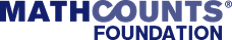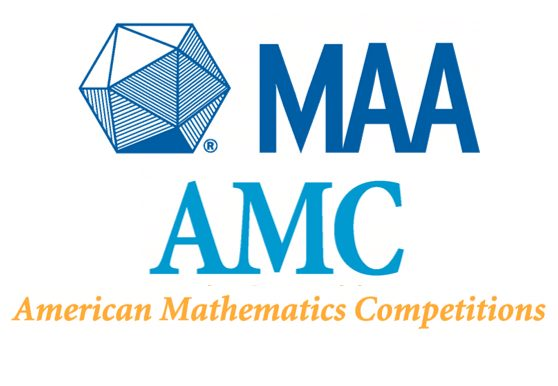Math Kangaroo Classes2018-05-22T20:57:09-04:00

Welcome to Math Kangaroo PrepMath Kangaroo ClassesImprove Math Kangaroo Scores with Accel Learning’s Math Kangaroo Prep Classes

Math Kangaroo is an international mathematical competition where over 50 countries are represented. There are twelve levels of participation, ranging from grade 1 to grade 12. The key competence tested by Mathematical Kangaroo is logical combination, not just pure knowledge of formulas. It is also the largest competition for school students in the world, with over 5,000,000 participants.

What is the structure of Math Kangaroo test?2017-10-17T20:44:31-04:00

What is the structure of Math Kangaroo test?

The Math Kangaroo tests are by grade pairs – so Grade 1 and 2 have the same test, Grades 3 and 4 have the same test and so on… up to grade 12.

The questions are of varying levels of difficulty. Many questions are more logic than math.

Questions are of 3 levels – ranging from 3 points (easy)  to 4 points (medium) and 5 points (difficult).

All questions are multiple choice with 5 options.

Calculators are not allowed in the test.

When is the Math Kangaroo held?2017-10-17T20:43:08-04:00

When is the Math Kangaroo held?

The Math Kangaroo is held in the middle of March each year.

Where is the Math Kangaroo held?2017-10-17T20:27:50-04:00

Where is the Math Kangaroo held?

The Math Kangaroo is held at After school centers such as at Accel Learning. Check out the website of Math Kangaroo to find the nearest center.

How long is the Math Kangaroo?2017-10-17T20:52:23-04:00

How long is the Math Kangaroo?

The Math Kangaroo consists of a series of about 24 questions to be answered in 75 minutes.

Where can I try out a sample the Math Kangaroo test?2017-10-17T20:26:58-04:00

Where can I try out a sample the Math Kangaroo test?

You can contact us at Accel Learning to try out a sample Math Kangaroo test.

How do I register for the Math Kangaroo?2017-10-17T20:21:26-04:00

How do I register for the Math Kangaroo?

The Math Kangaroos are held at local schools as well as at After school centers such as at Accel Learning. So you need to check with your school or contact us for registering for the Math Kangaroo.

Note, that you must register for the Math Kangaroo before December 31st to participate in March of the following year.

Where can I get more information about the Math Kangaroo?2017-10-17T20:16:44-04:00

ACCEL LEARNING